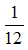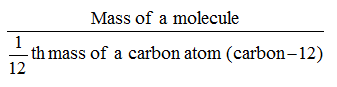Ex﻿

# 3. Atoms & Molecules

#### Molecular mass/Formula mass

Molecular mass expresses as to how many times a molecule of a substance is heavier thanth of the mass of an atom of carbon (carbon-12). Thus,
Molecular mass =Molecular mass = 2 × vapour density
Example A molecule of water is 18 times heavier thanth of the mass of carbon atom. Therefore, the molecular mass of water is 18 u.
Calculation of molecular mass from atomic masses
The molecules are made up of two or more atoms of different elements. Therefore, the molecular mass may be calculated as the sum of the atomic masses of all the atoms in a molecule of that substance.
Example. Ammonia has the formula, NH3. It consists of one atom of N and three atoms of H. The atomic mass of N and H are 14.0 and 1 respectively. Therefore, the molecular mass of NH3 is
Molecular mass of NH3 = At. mass of N + 3 × At. mass of H
= 14 + 3 × 1 = 17 u
Example. Sulphuric acid has the formula H2SO4. It consists of two H, one S and four O atoms. The atomic masses of H, S and O are 1,32 and 16 respectively. Therefore, the molecular mass of H2SO4 is
Molecular mass of H2SO4 =
(2 × at. m. of H) + (1 × at. m. of S)
+ (4 × at. m. of O)
= (2 × 1) + (1 × 32) + (4 × 16) = 98 u
Gram molecular mass
The molecular mass of a substance expressed in gram is called its gram molecular mass.
Example. Molecular mass of oxygen, O2 = 32 u
So, gram molecular mass of oxygen, O2 = 32 grams.

If you want to give information about online courses to other students, then share it with more and more on Facebook, Twitter, Google Plus. The more the shares will be, the more students will benefit. The share buttons are given below for your convenience.
×

#### NTSE Chemistry (Class X)

• Metals & Non Metals
• Elementary Idea of Bonding
• Chemical Reactions
• Acid Bases & Salts
• Compounds of Common Use
• Carbon and Its Compound
• Periodic Classification of Elements

#### NTSE Chemistry (Class IX)

• Matter in our Surrounding
• Is Matter Around us Pure
• Atoms & Molecules
• Structure of Atoms

SHOW CHAPTERS